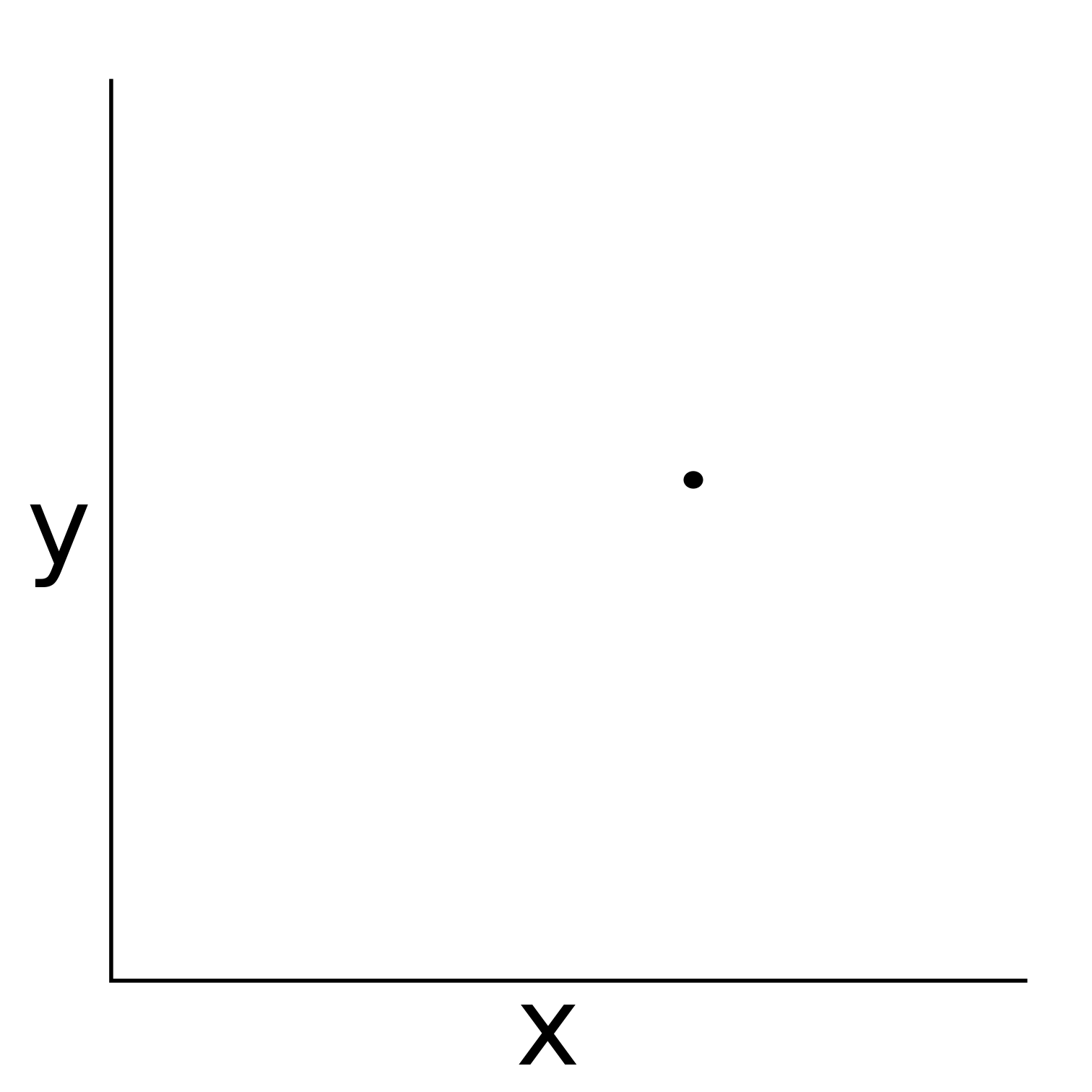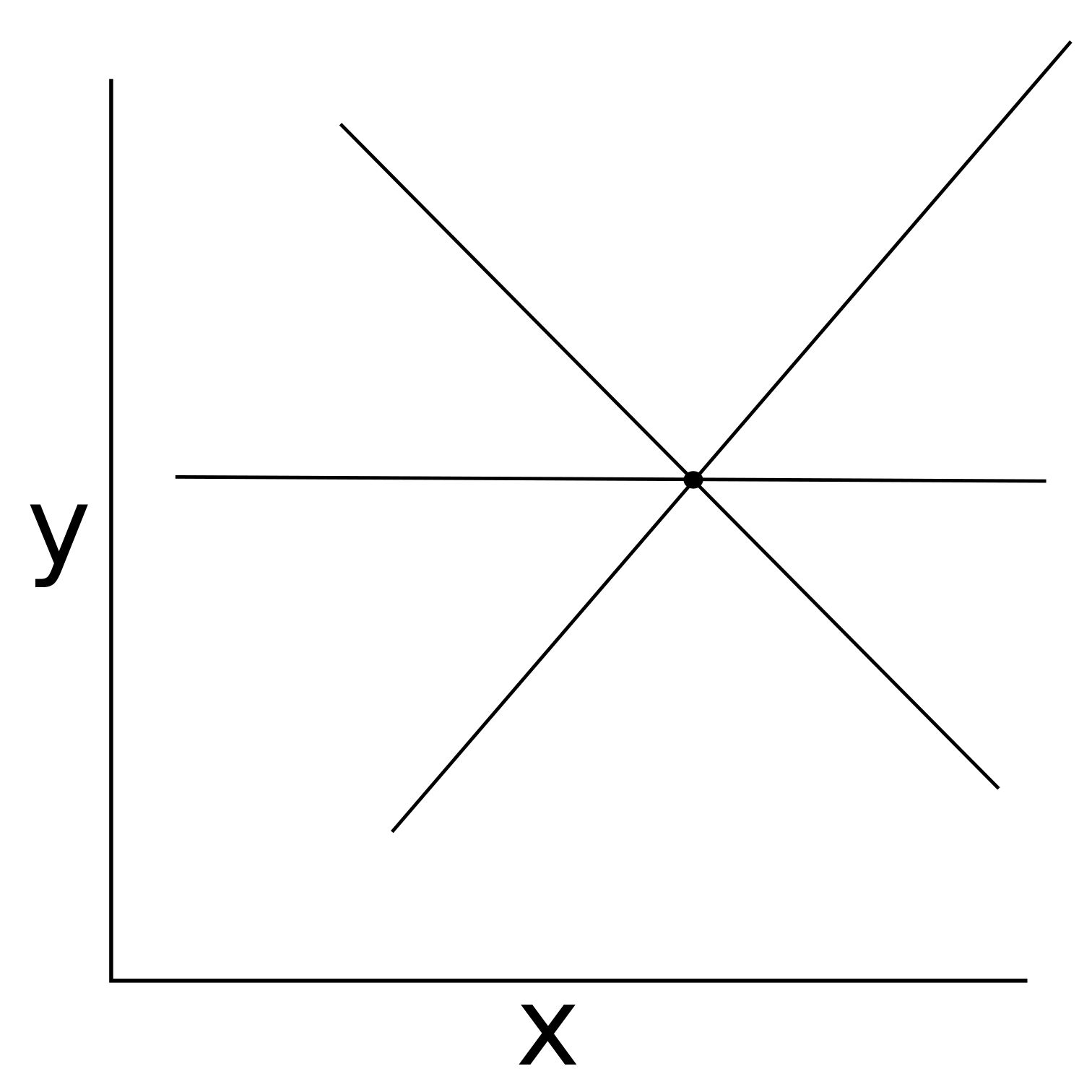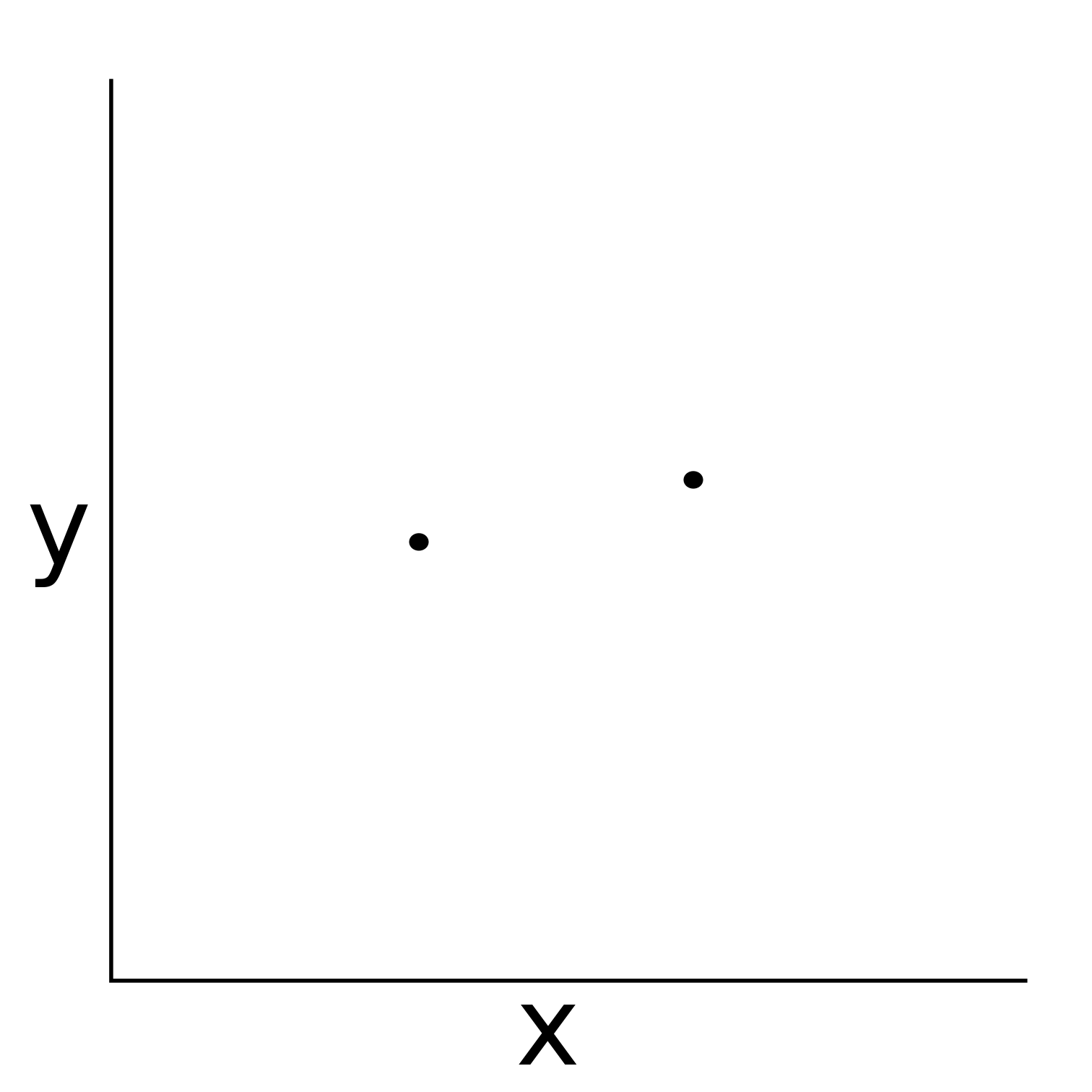# Regression part I

## Instructions:

Use the left and right arrow keys to navigate the presentation forward and backward respectively. You can also use the arrows at the bottom right of the screen to navigate with a mouse.

FAIR USE ACT DISCLAIMER:
This site is for educational purposes only. This website may contain copyrighted material, the use of which has not been specifically authorized by the copyright holders. The material is made available on this website as a way to advance teaching, and copyright-protected materials are used to the extent necessary to make this class function in a distance learning environment. The Fair Use Copyright Disclaimer is under section 107 of the Copyright Act of 1976, allowance is made for “fair use” for purposes such as criticism, comment, news reporting, teaching, scholarship, education and research.

## Outline

• The following topics will be covered in this lecture:
• An introduction to regression
• Simple linear regression
• Multiple linear regression
• Least squares solution
• Basic hypothesis testing
• Multiple regression in R
• Goodness of fit

## Introduction to regression

• Regression models are extremely important in describing relationships between variables.

• Linear regression is a simple, but powerful tool in investigating linear dependencies.

• It relies, however, on strict distributional assumptions in terms of how the relationship varies with respect to the regressors.
• Nonparametric regression models are widely used, because fewer assumptions about the data at hand are necessary.

• At the beginning of every empirical analysis, it is better to look at the data without assumptions about the family of distributions.

• Nonparametric techniques allow describing the observations and finding suitable models, when the sample size is sufficiently large and representative to explain the true population.

### Introduction to regression

• Regression models aim to find the most likely values of a dependent variable $$Y$$ for a set of possible values $$\{x_i\}_{i = 1}^n$$ of the explanatory variable $$X$$.

• We write a proposal for how the variables $$Y$$ and $$X$$ vary together as

\begin{align} Y = g(X) + \epsilon & & \epsilon \sim F_\epsilon , \end{align}

• where $$g(X)= \mathbb{E}\left[Y \vert X =x \right]$$ is an arbitrary function.

• The $$g(X)$$ is included in the model with the intention of capturing the mean of the process that corresponds to a particular value of $$X=x$$.

• If we believed that $$Y$$ had no dependency on the value of $$X=x$$, we could simply model this with respect to the average of the measured $$Y$$.
• The $$\epsilon$$ is a random noise term, representing variation around the deterministic part of the relationship.

• The natural aim is to keep the values of the $$\epsilon$$ as small as possible;

• that is to reduce the overall variation around the signal so that $$g(X)$$, the systematic part, explains as much of the relationship as possible.
• Parametric models assume that the dependence of $$Y$$ on $$X$$ can be fully explained by a finite set of parameters and that $$F_\epsilon$$ has a prespecified form with parameters to be estimated.

### Introduction to regression

• Nonparametric methods do not assume any form:

• neither for $$g(X)$$ nor for $$F_\epsilon$$, which makes them more flexible than the parametric methods.
• The fact that nonparametric techniques can be applied where parametric ones are inappropriate prevents the nonparametric user from employing a wrong method.

• These methods are particularly useful in fields like quantitative finance, where the underlying distribution is in fact unknown.

• However, as fewer assumptions can be exploited, this flexibility comes with the need for more data.

• Particularly, nonparametric methods can be of high variance in how they estimate the trend in the data.

• This can leave the methods susceptible to overfitting when the sample size is not large enough to differentiate the noise due to sampling error versus the true population level trend.

## Introducing linear models• In past mathematics courses, we have seen many examples of linear models.
• Suppose that we wish to model a relationship between two variables, $$x$$ and $$y$$ to the left.
• We will call $$y$$ a dependent variable, or the response variable.
• On the other hand, we will call $$x$$ an independent variable, an explanatory variable or a predictor variable for the response.
• Q: can you propose a valid linear model for the relationship between the response and the predictor?

### Introducing linear models – continued• A: actually, any line that passes through the point is a valid linear model.
• Particularly, this relationship is underconstrained and there exists infinitely many choices of linear models;
• given the current data, any choice is as valid as any other.

### Introducing linear models – continued• Q: given the data on the left, can you propose a valid linear model for the relationship between $$x$$ and $$y$$?